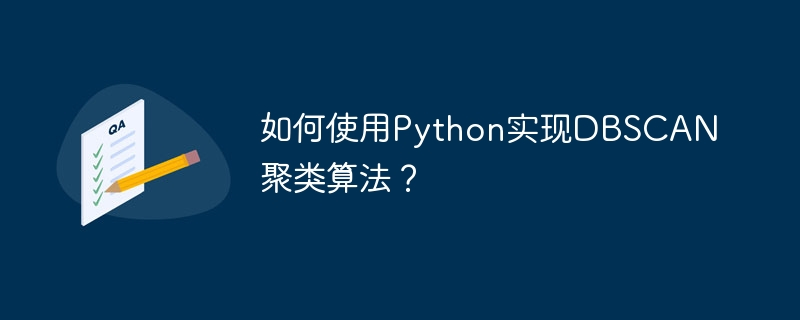# 如何使用Python实现DBSCAN聚类算法？WBOY 原创
2023-09-19 14:39:11 459浏览DBSCAN（Density-Based Spatial Clustering of Applications with Noise）是一种基于密度的聚类算法，可以自动识别具有相似密度的数据点，将它们划分为不同的簇。相比于传统的聚类算法，DBSCAN在处理非球形、不规则形状的数据集上表现出更高的灵活性和鲁棒性。本文将介绍如何使用Python实现DBSCAN聚类算法，并提供具体的代码示例。

1. 安装所需的库

```pip install numpy
pip install scikit-learn```
1. 导入所需的库和数据集

```import numpy as np
from sklearn.datasets import make_moons
from sklearn.cluster import DBSCAN

# 导入数据集
X, _ = make_moons(n_samples=200, noise=0.05, random_state=0)```
1. 创建DBSCAN对象并进行聚类

```# 创建DBSCAN对象
dbscan = DBSCAN(eps=0.3, min_samples=5)

# 对数据进行聚类
labels = dbscan.fit_predict(X)```
1. 可视化聚类结果

```import matplotlib.pyplot as plt

# 绘制聚类结果
plt.scatter(X[:,0], X[:,1], c=labels)
plt.xlabel("Feature 1")
plt.ylabel("Feature 2")
plt.title("DBSCAN Clustering")
plt.show()```

```import numpy as np
from sklearn.datasets import make_moons
from sklearn.cluster import DBSCAN
import matplotlib.pyplot as plt

# 导入数据集
X, _ = make_moons(n_samples=200, noise=0.05, random_state=0)

# 创建DBSCAN对象
dbscan = DBSCAN(eps=0.3, min_samples=5)

# 对数据进行聚类
labels = dbscan.fit_predict(X)

# 绘制聚类结果
plt.scatter(X[:,0], X[:,1], c=labels)
plt.xlabel("Feature 1")
plt.ylabel("Feature 2")
plt.title("DBSCAN Clustering")
plt.show()```